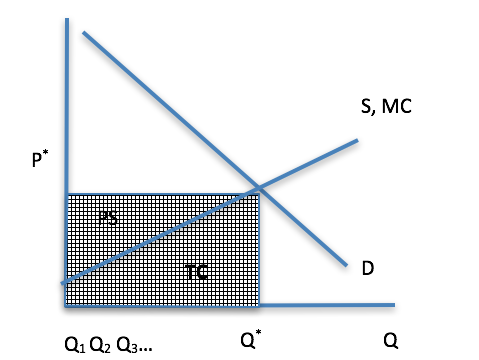# What Else Can We Determine from the Supply Side of the Market Model?

The answer is, lots! The position and slope of the curve provide information as does price and quantity and the areas under the curves. Let’s start with something you are already familiar with – total revenue. We know from a former module that price multiplied by quantity gives us revenue. Looking at Figure 7-4 below, you can see the same is true – TR is the area of the shaded rectangle.Figure 7-4: Total revenue, total cost and producer's surplus. Permission: Courtesy of course author Hayley Hesseln, Department of Agriculture and Resource Economics, University of Saskatchewan.

The hashed area represents total revenue, which is a transfer of money from the consumers who purchased Q* goods at price P*. But we know that not all the money is profit. Producers had to incur costs to produce those goods, and we can read that from the supply curve, also known as marginal cost.

The way to interpret marginal cost is by its looking at its definition – the cost of producing one more unit. If we look at the cost of unit #1, the cost is fairly low. The cost of unit #2 is a bit higher… and so on until we reach an equilibrium where the marginal cost of the last unit sold is equal to the price. If we add up the cost of producing each unit from 1 to Q* as read from the MC curve, we would have the total cost, which is the area under the marginal cost curve.

That leaves only one small shaded area not accounted for – the area above the MC curve. We can calculate that as TR – TC = Producer surplus. When we calculated break-even analysis, we called this value, “profit.” It is in fact profit, also known as “rent,” and producer surplus that accrues to the producer for his or her entrepreneurial talents.Source: http://www.usnews.com/news/us/articles/2013/01/12/with-almonds-rising-revenues-land-values-soar Permission: This material has been reproduced in accordance with the University of Saskatchewan interpretation of Sec.30.04 of the Copyright Act.